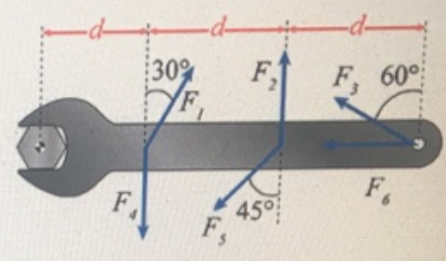# Problem: Problem 6: A wrench is used to apply a torque to a bolt. Forces F, through F6 all have the same magnitude. The point of application of force F, (and F) is a distance d from the center of rotation of the bolt. The application points of F, F2, and F3 are all distance d apart, as shown. Use the convention that counter-clockwise torques are positive; clockwise torques are negative.Part (a) Determine the torque applied on the bolt by F4 in terms of the variables and angles given in the problem.Part (b) Determine the torque applied on the bolt by F5 in terms of the variables and angles given in the problem.Part (c) Determine the torque applied on the bolt by F6 in terms of the variables and angles given in the problem.

###### FREE Expert Solution

Torque:

$\overline{){\mathbf{\tau }}{\mathbf{=}}{\mathbf{r}}{\mathbf{F}}{\mathbf{s}}{\mathbf{i}}{\mathbf{n}}{\mathbf{\theta }}}$

where r is the position vector and θ is the angle between the force vector and the lower arm vector.

97% (50 ratings)###### Problem Details

Problem 6: A wrench is used to apply a torque to a bolt. Forces F, through F6 all have the same magnitude. The point of application of force F, (and F) is a distance d from the center of rotation of the bolt. The application points of F, F2, and F3 are all distance d apart, as shown. Use the convention that counter-clockwise torques are positive; clockwise torques are negative.Part (a) Determine the torque applied on the bolt by F4 in terms of the variables and angles given in the problem.

Part (b) Determine the torque applied on the bolt by F5 in terms of the variables and angles given in the problem.

Part (c) Determine the torque applied on the bolt by F6 in terms of the variables and angles given in the problem.